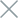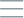• Home
• Tags
• Series
•# Random Search Algorithm in C++ - Explanation, Example and Code

The Random Search algorithm is a search method based on randomly selecting a set of solutions from the search space and checking if they can solve the problem. This approach is often used when there is no specific information or strategy to guide the search.

## How It Works

2. Evaluation: Evaluate the quality of each solution based on the objective function or evaluation criteria.
3. Selection: Select a subset of the best solutions from the set based on probabilities or random selection.
4. Testing: Test if the selected solutions are capable of solving the problem.
5. Repeat: Iterate through steps 2 to 4 until a satisfactory result is achieved or a predefined number of iterations is reached.

## Example: Optimizing the Fibonacci Function

Consider the optimization problem of the Fibonacci function F(x) = F(x-1) + F(x-2) with F(0) = 0, F(1) = 1. We want to find the value of x for which F(x) is maximized. The Random Search method can randomly select values of x, calculate the Fibonacci value at each x, and choose the value of x corresponding to the highest Fibonacci value obtained.

## Code Example in C++

``````#include <iostream>
#include <cstdlib>
#include <ctime>

int fibonacci(int n) {
if (n <= 0) return 0;
if (n == 1) return 1;
return fibonacci(n - 1) + fibonacci(n - 2);
}

int randomSearchFibonacci(int maxIterations) {
int bestX = 0;
int bestValue = 0;

srand(time(0));

for (int i = 0; i < maxIterations; ++i) {
int x = rand() % maxIterations;
int value = fibonacci(x);
if (value > bestValue) {
bestValue = value;
bestX = x;
}
}

return bestX;
}

int main() {
int maxIterations = 20;
int result = randomSearchFibonacci(maxIterations);

std::cout << "Optimal x for maximum Fibonacci value: " << result << std::endl;

return 0;
}
``````

In this example, we use the Random Search method to optimize the Fibonacci function. We randomly select values of x, calculate the Fibonacci value at each x, and then choose the value of x corresponding to the highest Fibonacci value we have calculated.